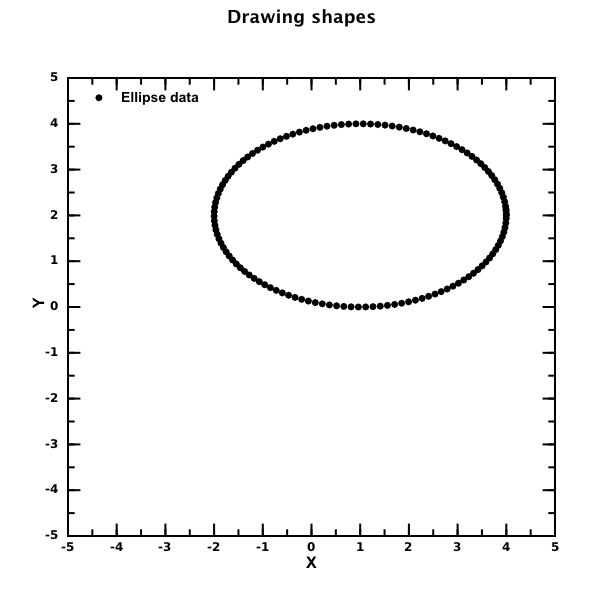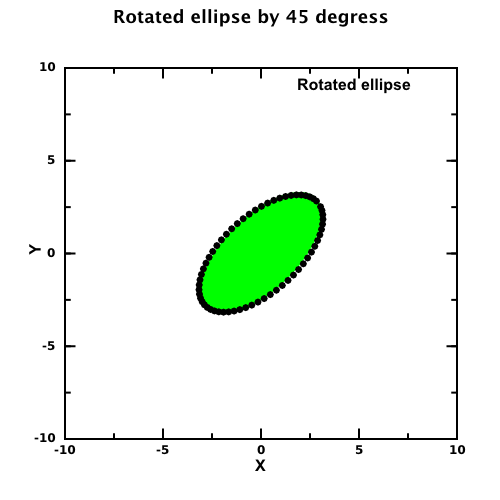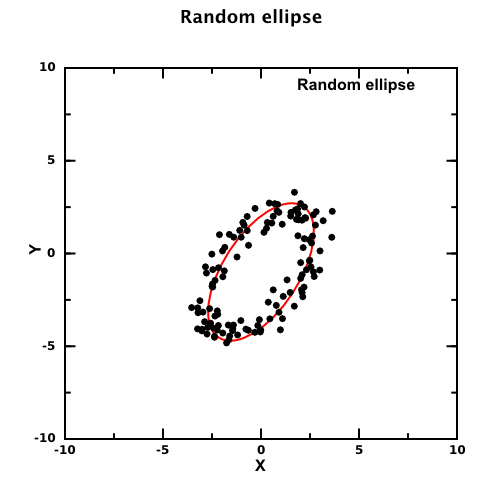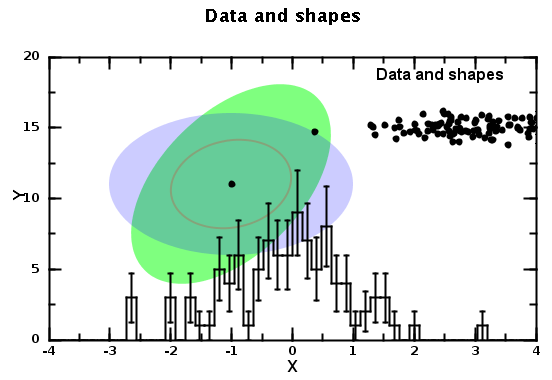# DMelt:Plots/4 Showing 2D Shapes

Limitted access. First login to DataMelt if you are a full DataMelt member. Then login to HandWiki as a user.

# Simple 2D shapes

In addition to data, histograms, functions,jhplot.HPlot canvas can show arbitrary shapes (boxes, text, images, arrows) in the user ("USER") coordinates (i.e. given by the axis values) or in the absolute coordinates ("NDC"). You can mix data and shapes in arbitrary order.

The following shapes can be plotted:

You should also remember that you can always draw shapes with data points. Here is an example how to draw an ellipse with semi-major (longest radius) and semi-minor (shortest radius) radii:Here is a simple code to do this:

You can convert it to Groovy or Java code using the same logic. Decrease the step size to obtain a smooth line.

Now let us construct rotated ellipse by some angle and overlay it with a 2D Ellipse using "2D" shape approach:The code to generate this image is below:

Finally, one can generate random data points following the ellipse shape. We will use parametric description of the ellipse, rotate it and generate random numbers:The Python code this image is below:

You can use the basic 2D primitives to draw shapes. Here is a simple example how to draw such shapes:You can find a script that generates this image below. It shows how to add X-Y data, histograms a then draw simple 2D graphic primitives on the top: